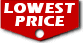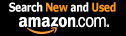# Partial Differential Equations for Scientists and Engineers (Dover Books on Mathematics) / Stanley J. Farlow

List Price: \$18.95
Our Price: \$18.95For Bulk orders
Quantity

Used Book Price:
/ Stanley J. Farlow
Publisher: Dover Publications
Availability:In Stock.
Sales Rank: 60837
 ISBN-10: 048667620XISBN-13: 9780486676203

Similar Books
 Ordinary Differential Equations (Dover Books on Mathematics) Fourier Series (Dover Books on Mathematics) Schaum's Outline of Partial Differential Equations (Schaum's Outlines) Linear Algebra (Dover Books on Mathematics) Schaum's Outline of Differential Equations, 4th Edition (Schaum's Outlines) Introduction to Partial Differential Equations with Applications (Dover Books on Mathematics) Numerical Methods for Scientists and Engineers (Dover Books on Mathematics) A Student's Guide to Maxwell's Equations (Student's Guides) An Introduction to Information Theory: Symbols, Signals and Noise (Dover Books on Mathematics) Introduction to Topology: Third Edition (Dover Books on Mathematics)

Most physical phenomena, whether in the domain of fluid dynamics, electricity, magnetism, mechanics, optics, or heat flow, can be described in general by partial differential equations. Indeed, such equations are crucial to mathematical physics. Although simplifications can be made that reduce these equations to ordinary differential equations, nevertheless the complete description of physical systems resides in the general area of partial differential equations.
This highly useful text shows the reader how to formulate a partial differential equation from the physical problem (constructing the mathematical model) and how to solve the equation (along with initial and boundary conditions). Written for advanced undergraduate and graduate students, as well as professionals working in the applied sciences, this clearly written book offers realistic, practical coverage of diffusion-type problems, hyperbolic-type problems, elliptic-type problems, and numerical and approximate methods. Each chapter contains a selection of relevant problems (answers are provided) and suggestions for further reading.

Now you can buy Books online in USA,UK, India and more than 100 countries.
*Terms and Conditions apply
Disclaimer: All product data on this page belongs to.
No guarantees are made as to accuracy of prices and information.